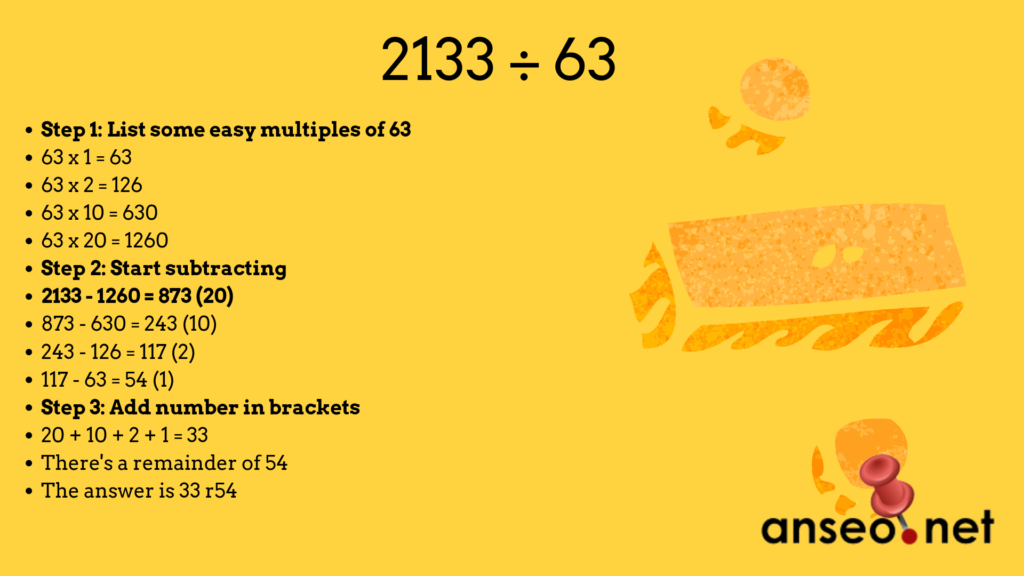## 088. Lose Long Division from the Maths CurriculumGenerally, most of these ideas are quite critical of the Department of Education. However, one thing they got right, generally, was the Maths Curriculum in 1999.

Fast forward almost 2 decades and the government are trying to undo all their good work with a new curriculum based on the failed Primary Language Curriculum. Anyway, this post isn’t about that – it’s about the only weak point in the 1999 curriculum – Long Division.

If you look at all other aspects of the curriculum, you would be able to teach them using concrete objects and pictorial representations. However, when it comes to Long Division, you basically have to teach a method – Divide, Multiply, Subtract, Bring Down. There’s no visual way to explain why to do this (at least not to primary school kids.)

Sure, there’s ways to remember the routine – Does Messi Support Barcelona? Does McDonald’s Sell Burgers? However, these don’t explain the mathematics, they just might help a child remember the order of the routine.

My suggestion is to scrap it from the curriculum and replace it with a version of repeated subtraction, because this makes sense. Below is an image of how to do “Super Repeated Subtraction” for a random division sum 2133 ÷ 63The idea here is that division is simply a matter of repeating a subtraction. Multiplying the divisor helps speed things up as can be seen above. It works nicely and can be explained to any pupil.

Using the same method above let’s try 6,205 ÷ 95

• 95 x 1 = 95
• 95 x 2 = 190
• 95 x 10 = 950
• 95 x 20 = 1900

So let’s go

• 6205 – 1900 = 4305 (20)
• 4305 – 1900 = 2405 (20)
• 2405 – 1900 = 505 (20)
• 505 – 190 = 315 (2)
• 315 – 190 = 125 (2)
• 125 – 95 = 30 (1)

So adding the bracketed numbers means we get the answer 65 r:30

## Related Articles

• Search Knowledgebase